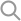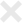The hyperparameter tuning module allows us to select the optimal engine parameter defined by a Metric. Metric determines the quality of an engine variant. We have skimmed through the process of choosing the right Metric in previous sections.

This section discusses basic evaluation metrics commonly used for classification problems. If you are more interested in knowing how to implement a custom metric, please skip to the next section.

## Defining Metric

Metric evaluates the quality of an engine by comparing engine's output (predicted result) with the original label (actual result). A engine serving better prediction should yield a higher metric score, the tuning module returns the engine parameter with the highest score. It is sometimes called loss function in literature, where the goal is to minimize the loss function.

During tuning, it is important for us to understand the definition of the metric, to make sure it is aligned with the prediction engine's goal.

In the classification template, we use Accuracy as our metric. Accuracy is defined as: the percentage of queries which the engine is able to predict the correct label.

## Common Metrics

We illustrate the choice of metric with the following confusion matrix. Row represents the engine predicted label, column represents the actual label. The second row means that of the 200 testing data points, the engine predicted 60 (15 + 35 + 10) of them as label 2.0, among which 35 are correct prediction (i.e. actual label is 2.0, matches with the prediction), and 25 are wrong.

Actual = 1.0 Actual = 2.0 Actual = 3.0
Predicted = 1.0 30 0 60
Predicted = 2.0 15 35 10
Predicted = 3.0 0 0 50

### Accuracy

Accuracy means that how many data points are predicted correctly. It is one of the simplest form of evaluation metrics. The accuracy score is # of correct points / # total = (30 + 35 + 50) / 200 = 0.575.

### Precision

Precision is a metric for binary classifier which measures the correctness among all positive labels. A binary classifier gives only two output values (i.e. positive and negative). For problem where there are multiple values (3 in our example), we first have to transform our problem into a binary classification problem. For example, we can have problem whether label = 1.0. The confusion matrix now becomes:

Actual = 1.0 Actual != 1.0
Predicted = 1.0 30 60
Predicted != 1.0 15 95

Precision is the ratio between the number of correct positive answer (true positive) and the sum of correct positive answer (true positive) and wrong but positively labeled answer (false positive). In this case, the precision is 30 / (30 + 60) = ~0.3333.

### Recall

Recall is a metric for binary classifier which measures how many positive labels are successfully predicted amongst all positive labels. Formally, it is the ratio between the number of correct positive answer (true positive) and the sum of correct positive answer (true positive) and wrongly negatively labeled answer (false negative). In this case, the recall is 30 / (30 + 15) = ~0.6667.

As we have discussed several common metrics for classification problem, we can implement them using the Metric class in the next section.